#### Howdy, Stranger!

It looks like you're new here. Sign in or register to get started.

# A Generalization of Lucas de Groot’s Interpolation Theory

After stumbling over the stem interpolation calculator http://typedrawers.com/discussion/205/family-stem-weights-calculator , I have realized that some of the wishes mentioned there (choose a third interpolation point, make interpolation value 0 possible) remembered me to interpolation problems I had some years ago (for the optical size axis instead of the weight axis). I have generalized Lucas de Groot's Interpolation Theory in order to get a solution for these wishes:

Lucas de Groot’s Interpolation Theory states, that font weights of a family of fonts should progress like a geometric sequence. E.g. the first font should have the weight 20 (denoted as A(1, 20) in the image below left) and the ninth font should have the weight 220 (denoted as B(9, 220) and the weights inbetween develop as depicted by the graph. In mathematical terms Lucas de Groot’s Interpolation Theory means interpolating the points A and B by an exponential function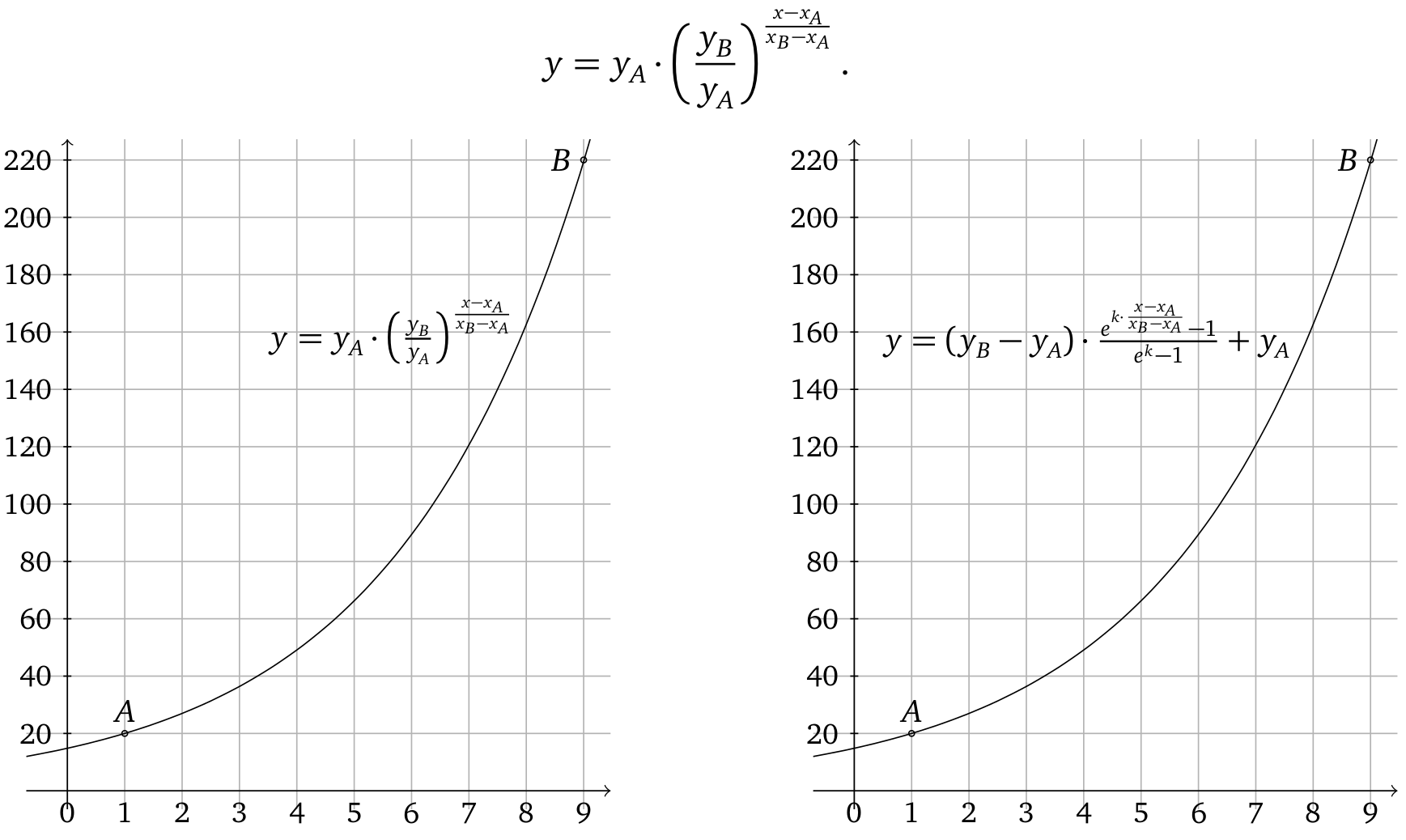This function is a special case of a generalized set of functions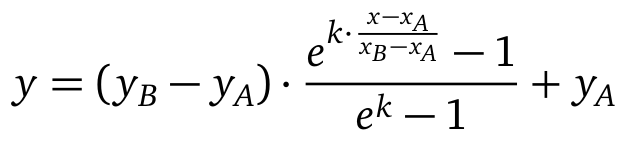depicted in the upper right. This means, when we choose the parameter k = ln(y_B/y_A) , the upper right function is the same as the upper left function. The great thing about this is, that we can vary now k in order to get similar curves (for k = 0 we have to use a linear function in order to prevent divison by zero):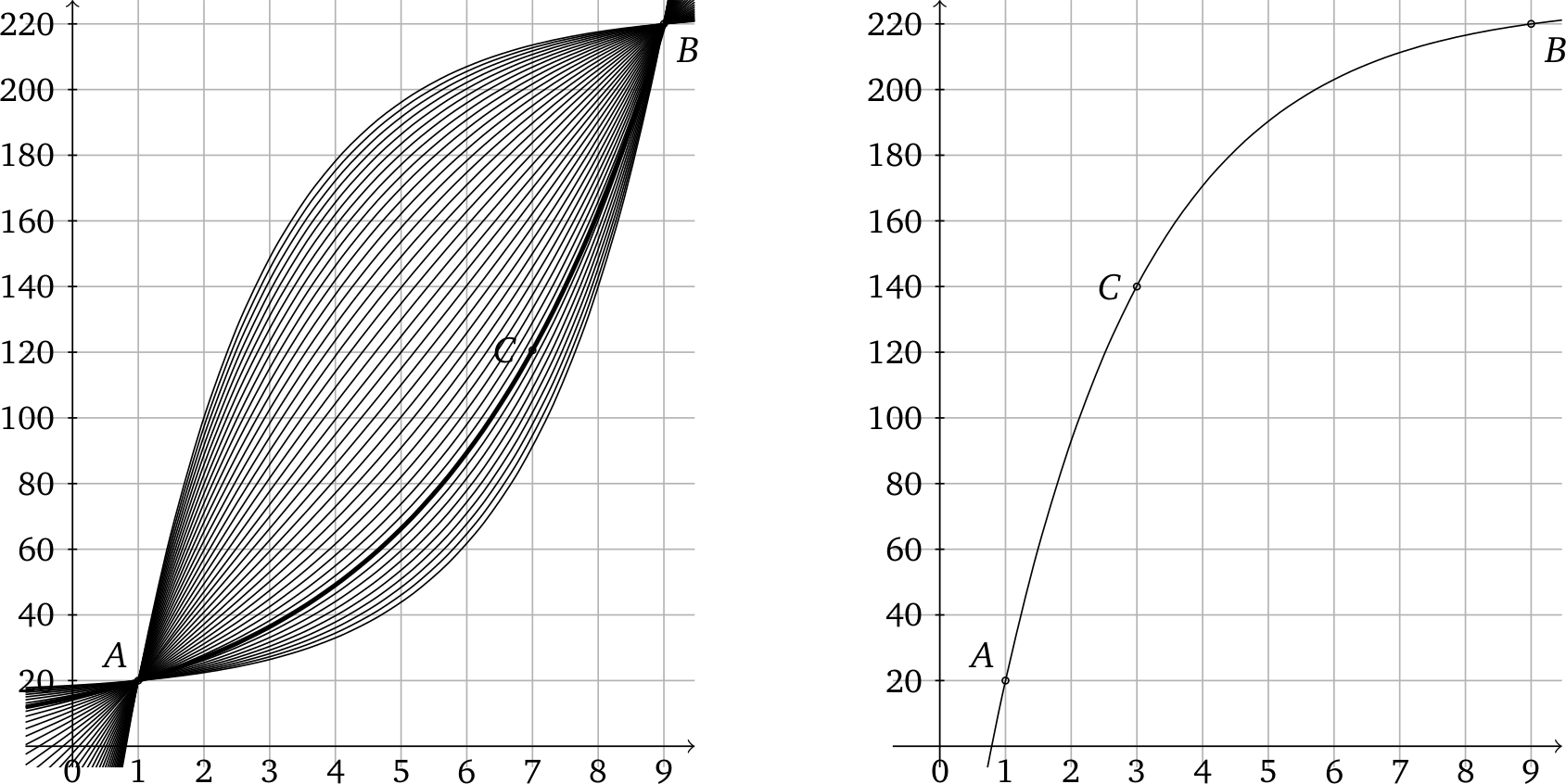With the generalized function, we can now interpolate three points A, B and C — as long as monotony is still given (depicted in the upper right). Of course, we lose the property of geometric progression. In return, it is now possible that y becomes zero.

I have written a Java program (rename the attached stepinterpolation.zip to stepinterpolations.jar and you have the program). That works either with Lucas de Groot's original interpolation or my generalized version depending on th input.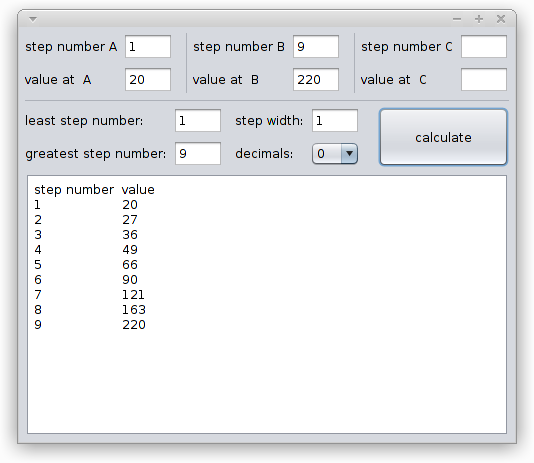If no point C is given, the values are Lucas de Groot's original interpolation. We can tweak the output by things like setting more decimals: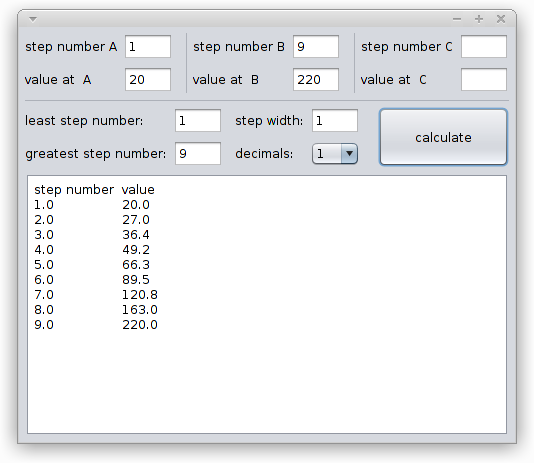Now, if we set the point C to an already existing value, nothing will change: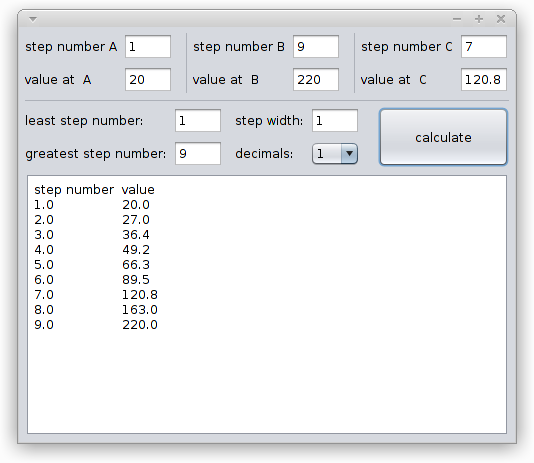Of course, this would not make much sense, but it shows, that putting a third point is kind of stable. Now we really  take a different point C and get different values (remember that we now have lost the property of geometric progression that makes up Lucas de Groot's Theory):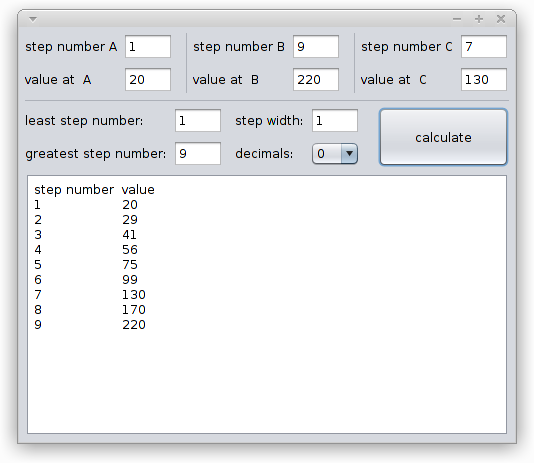We can also just take two values and set the value at A to zero: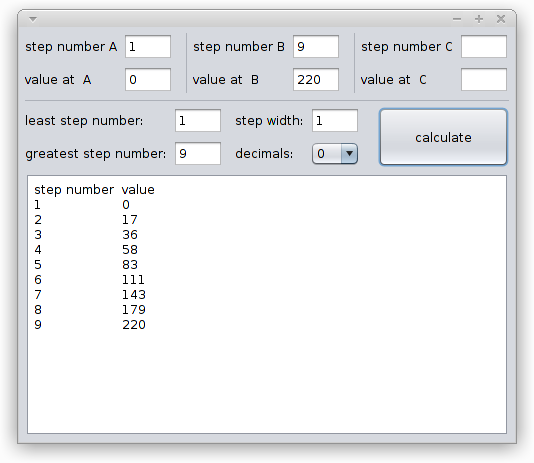Here is an example for choosing stem widths along an optical size axis (ranging from 6pt to 72pt):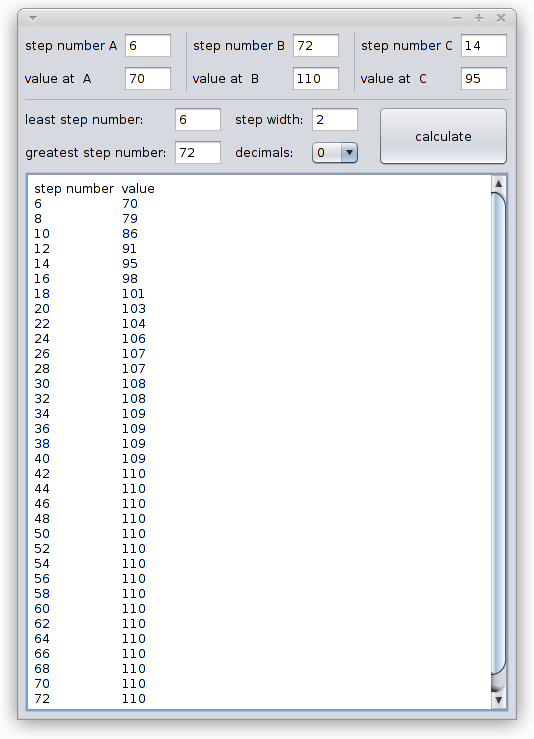This may also show, why additional sizes between 24pt and 72pt are not that interesting.

You can interpolate any two or three points (C is the optional point) as long as the points develop monotonous (i.e. values are strictly increasing or strictly falling). Have fun with the program!
Tagged:

• That's very interesting! Thanks for sharing that. I'm a little confused at how point C is accounted for in the equation. I can tell what it's supposed to do, but where do you put its values?
• Nevermind. I get it. You plug in the x,y values for point C into the equation and solve for k. Got it.
• edited April 2018
With the generalized function, we can now interpolate three points A, B and C

Interesting indeed. Being able to calculate interpolation values for three points, instead of two, is a valuable addition, in case one doesn’t want to use a De Groot progression. (When using a De Groot progression, it is possible to do two calculations for two points, with one of the points of each of those two calculations being the same point. Then the result will be a De Groot progression with three points.)

Of course, we lose the property of geometric progression. In return, it is now possible that y becomes zero.

Both this disadvantage and advantage are irrelevant. The moment one decides to use another progression than a De Groot progression, one decides to forgo geometric progression. A y value of zero does not exist in reality, because a glyph with a stem width of zero does not exist.

For me, it would be clearer if the names of the B and C points would be swapped, and if the current B point (the highest value) would be most to the right in the user interface of the program. Then A would be the smallest stem width, B would be an intermediate stem width, and C would be the biggest stem width.

It can be expected that in practice, there will be no values of the intermediate stem width above a straight line between the smallest stem width and the biggest stem width—so the last graph is not a practical example. [It would make sense if the program would block any value input for an intermediate stem width above a linear progression, or would give a warning in such cases. In addition, it may be helpful if the program would display a value for the degree of linearity of the progression which results from the user input—because then it will be possible (by trial and error) to replicate (as near as possible) the degree of linearity which has been used in one font family, in another font family.]

• Ben Blom said:
For me, it would be clearer if the names of the B and C points would be swapped, and if the current B point (the highest value) would be most to the right in the user interface of the program. Then A would be the smallest stem width, B would be an intermediate stem width, and C would be the biggest stem width.

[...]

In addition, it may be helpful if the program would display a value for the degree of linearity of the progression which results from the user input—because then it will be possible (by trial and error) to replicate (as near as possible) the degree of linearity which has been used in one font family, in another font family.]
Okay, done (see attachment, .zip has to be changed to .jar in order to run with Java).

The example with the optical sizes were the wrong way round, here is the correct version: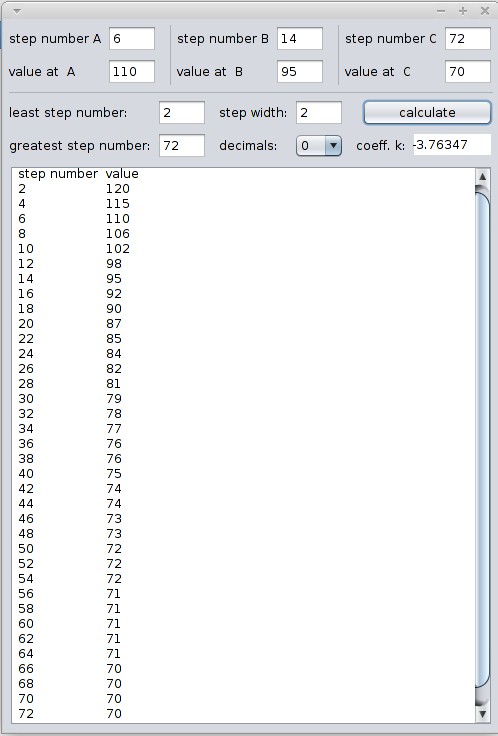Ben Blom said:

It can be expected that in practice, there will be no values of the intermediate stem width above a straight line between the smallest stem width and the biggest stem width—so the last graph is not a practical example. [It would make sense if the program would block any value input for an intermediate stem width above a linear progression, or would give a warning in such cases.

I think, above straight lines is not uncommon. Lucas de Groot anisotropic topology-dependent interpolation theory uses something similar (image is a link from http://www.lucasfonts.com/about/interpolation-theory/ ):With generalized functions: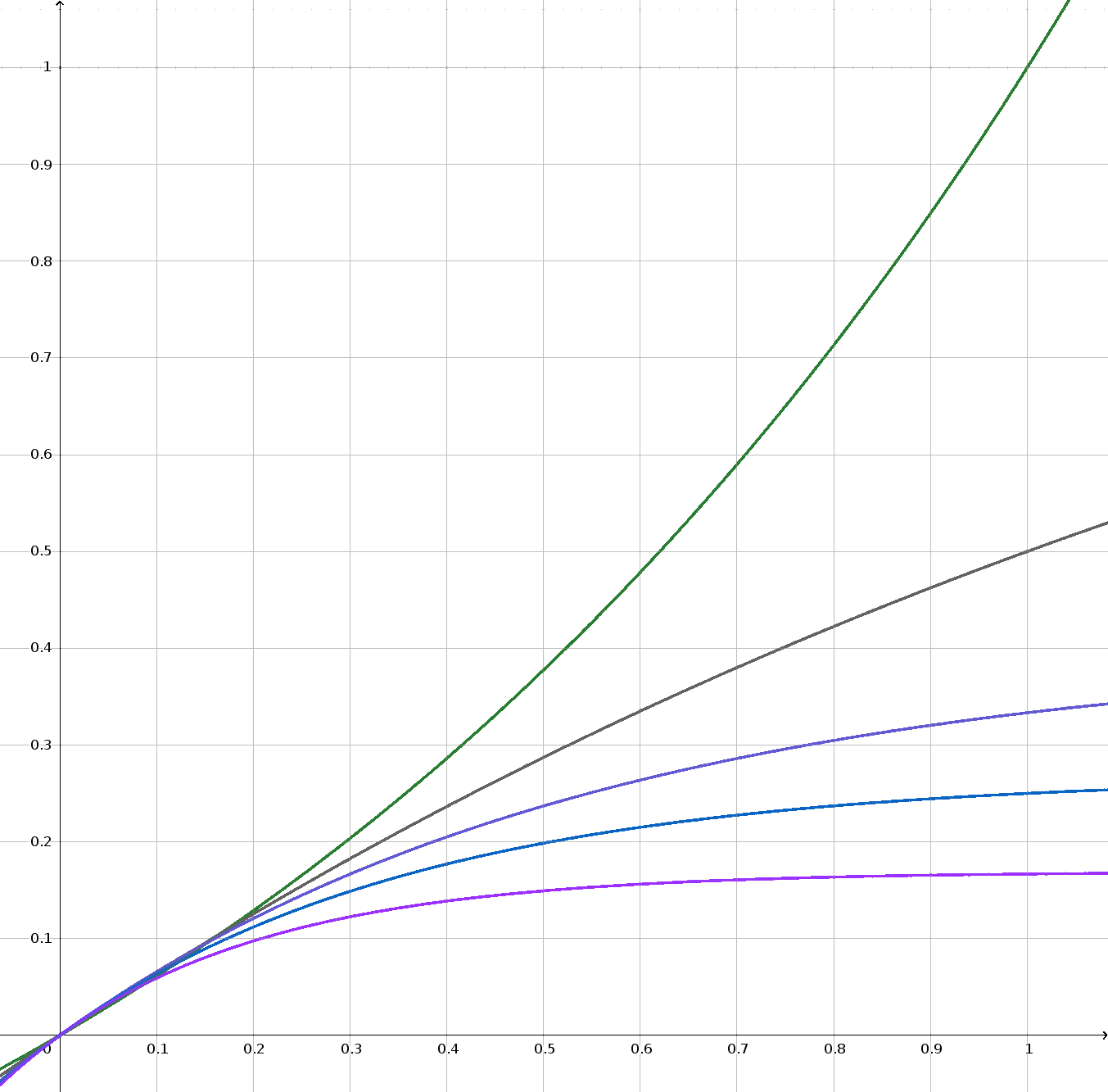• Linus, thank you for the changes in your program. Something seems to be wrong with your last user interface example: “Step number A” has value 6, and “least step number” has value 2. I would expect these values to be the same.

The image you took from De Groot, is from another theory of De Groot—not from what is usually understood as Lucas de Groot’s weight progression. De Groot’s weight progression is described in your source as: «the optically correct in-between weights are on a hollow curve that produces values which are lower than those on the straight line of the “average values”».

The usual weight progressions are below (and including) a straight line. See this image which belongs below “All this area is explorable” here, and see the image below “Take a look at this chart” here.

• @Ben Blom
Something seems to be wrong with your last user interface example: “Step number A” has value 6, and “least step number” has value 2. I would expect these values to be the same.
For most cases (and also for the given case), I would also expect these values to be the same (but the you have the freedom to change it):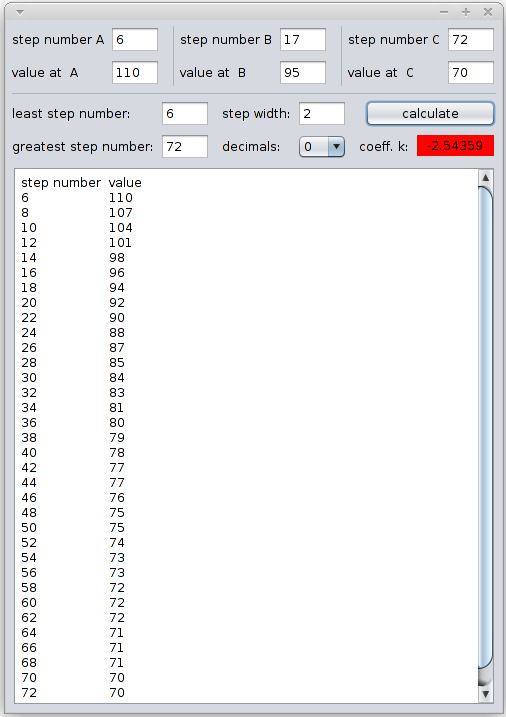You may have noticed, that I have made the background of the coefficient red as a warning for being out of the “All this area is explorable” (otherwise it will become green).
The image you took from De Groot, is from another theory of De Groot—not from what is usually understood as Lucas de Groot’s weight progression.
Yes, I tried to indicate clearly so in my post above. I would say Lucas de Groot anisotropic topology-dependent interpolation theory is a generalization of his older interpolation theory as the V-curve is based on it but the H-curves are not.

The usual weight progressions are below (and including) a straight line. See this image which belongs below “All this area is explorable” here, and see the image below “Take a look at this chart” here.
Thanks for the links, I could implement the Abraham curve in the other program here (at the end of the discussion):

http://typedrawers.com/discussion/205/family-stem-weights-calculator
• I have made github repositories for my program posted in this discussion and also for my other program posted in the discussion http://typedrawers.com/discussion/205/family-stem-weights-calculator .

Feel free to fork the programs:

stepinterpolation
https://github.com/linusromer/stepinterpolation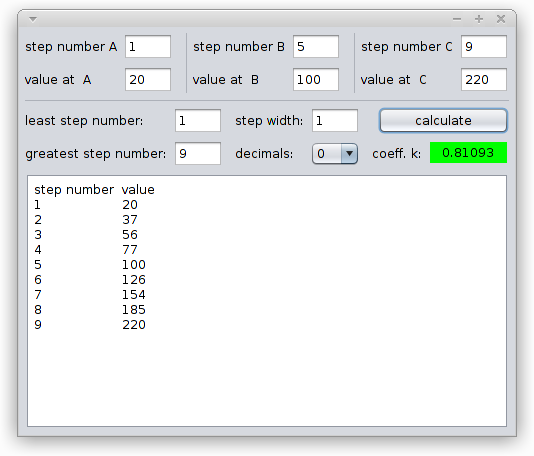Direct link to the executable JAR:
https://github.com/linusromer/stepinterpolation/blob/master/dist/stepinterpolation.jar

steminterpolation
https://github.com/linusromer/steminterpolation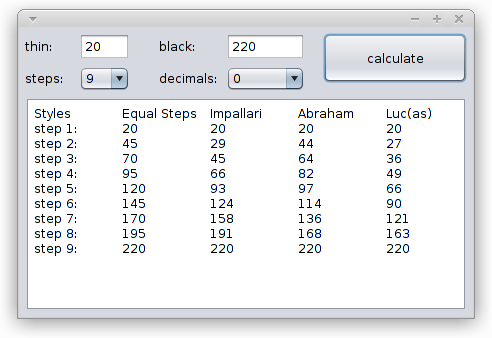Direct link to the executable JAR:
https://github.com/linusromer/steminterpolation/blob/master/dist/steminterpolation.jar
• Great license choice)